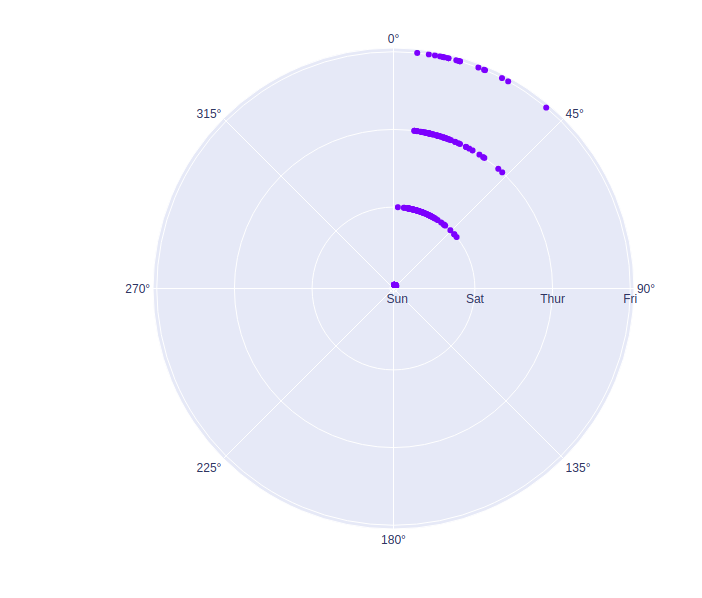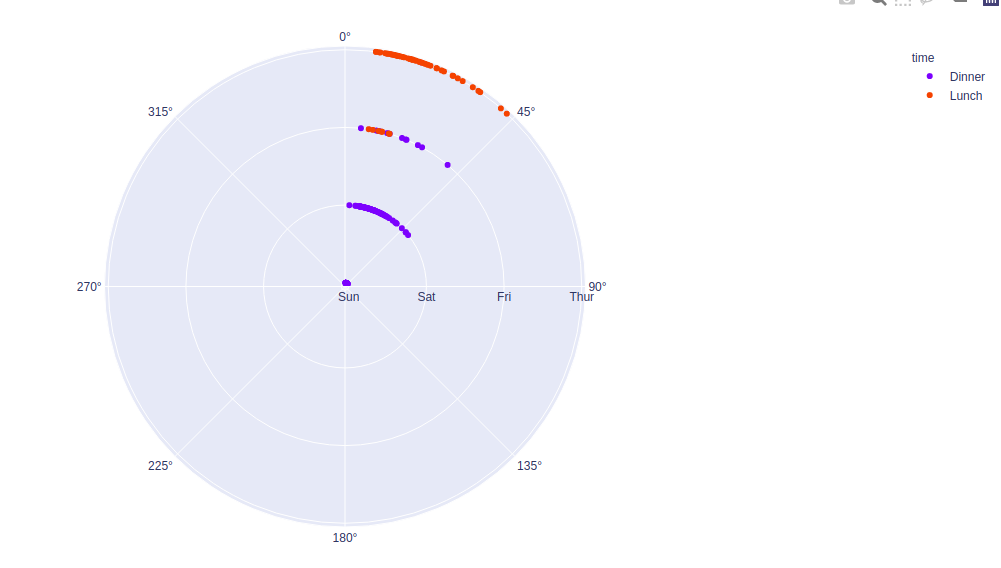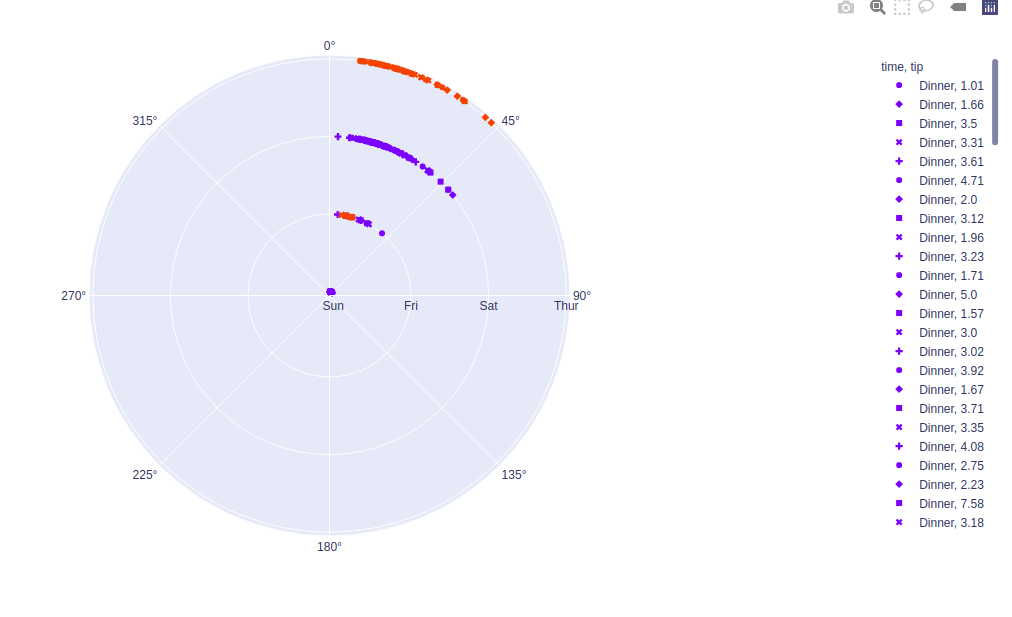Open in App
Not now

# plotly.express.scatter_polar() function in Python

• Last Updated : 17 Jul, 2020

Plotly library of Python can be very useful for data visualization and understanding the data simply and easily. Plotly graph objects are a high-level interface to plotly which are easy to use.

## plotly.express.scatter_polar() function

This function is used to create a polar chart. A polar chart represents data along radial and angular axes.

Syntax: plotly.express.scatter_polar(data_frame=None, r=None, theta=None, color=None, symbol=None, size=None, hover_name=None, hover_data=None, title=None, template=None, width=None, height=None)

Parameters:

data_frame: DataFrame or array-like or dict needs to be passed for column names.

r, theta: This parameter is either a name of a column in data_frame, or a pandas Series or array_like object. It is used to position marks along the radial and angular axis in polar coordinates respectively.

color: This parameters assign color to marks.

symbol: This parameter is used to assign symbols to marks. It is either a name of a column in data_frame, or a pandas Series or array_like object.

size: This parameter is used to assign mark sizes. It is either a name of a column in data_frame, or a pandas Series or array_like object.

hover_name:  Values from this column or array_like appear in bold in the hover tooltip.

hover_data: This parameter is used to appear in the hover tooltip or tuples with a bool or formatting string as first element, and list-like data to appear in hover as second element Values from these columns appear as extra data in the hover tooltip.

title: The figure title

width: The figure width in pixels.

height: The figure height in pixels.

Example 1:

## Python3

 `import` `plotly.express as px`` ` `df ``=` `px.data.tips()`` ` `plot ``=` `px.scatter_polar(df, r ``=` `'day'``, ``                        ``theta ``=` `'total_bill'``)``plot.show()`

Output:Example 2: Using color argument

## Python3

 `import` `plotly.express as px`` ` `df ``=` `px.data.tips()`` ` `plot ``=` `px.scatter_polar(df, r ``=` `'day'``,``                        ``theta ``=` `'total_bill'``, ``                        ``color``=``'time'``)``plot.show()`

Output:Example 3: Using symbol argument

## Python3

 `import` `plotly.express as px`` ` `df ``=` `px.data.tips()`` ` `plot ``=` `px.scatter_polar(df, r ``=` `'day'``, ``                        ``theta ``=` `'total_bill'``,``                        ``color ``=` `'time'``,``                        ``symbol ``=` `'tip'``)``plot.show()`

Output:My Personal Notes arrow_drop_up